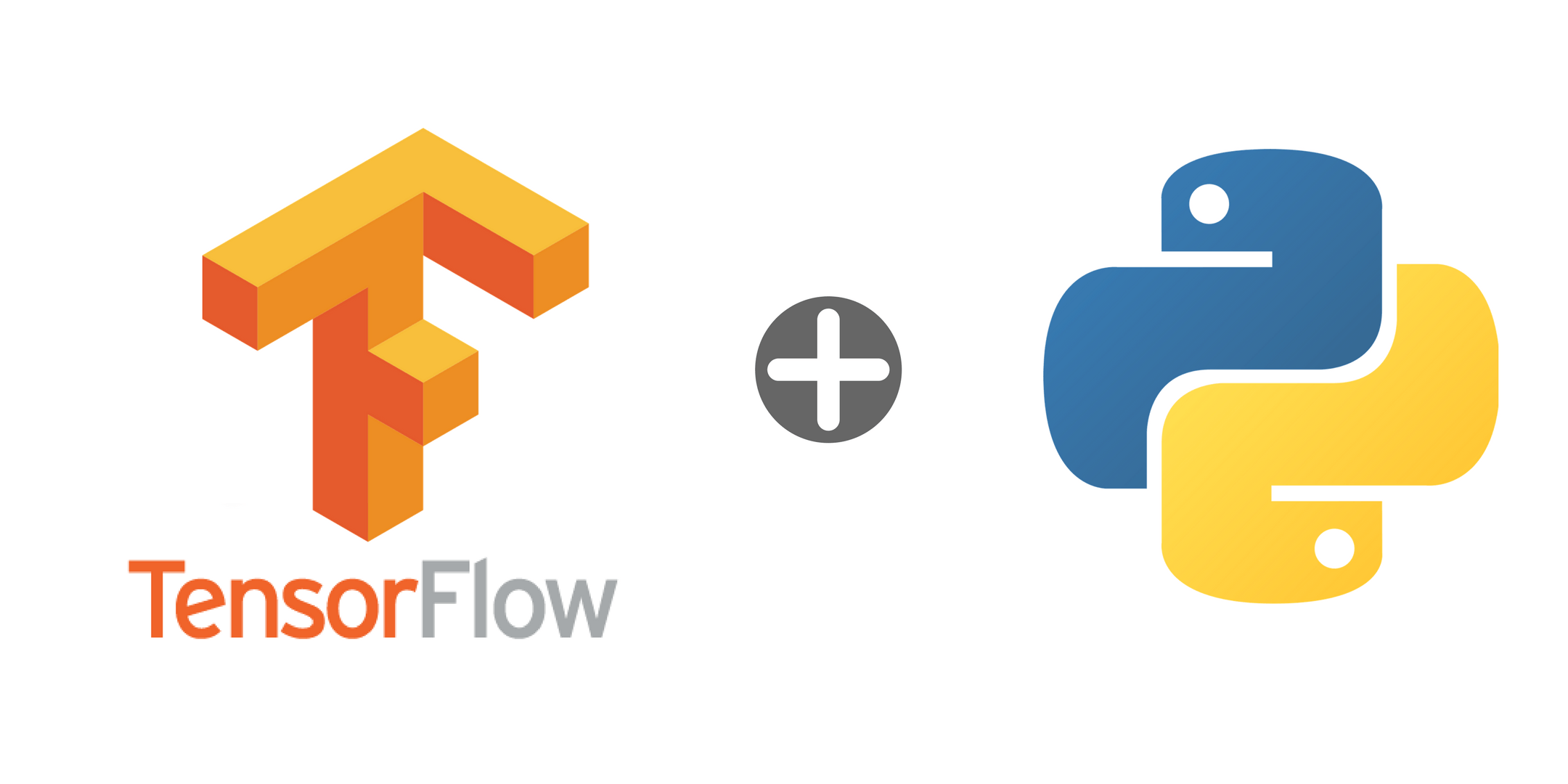# An Introduction to Tensorflow with Python

Artificial Intelligence, Machine Learning, and Deep Learning are very popular and important in the world of technology. There are few libraries in Python to work with these fields. TensorFlow is a very popular open source library used for deep learning. TensorFlow can be controlled by a simple Python API

TensorFlow is an open source library for fast numerical computing used both in research and development and in production systems. It is a symbolic math library used as a system for building and training neural networks to detect and decipher patterns and correlations. Tensorflow can perform computation very efficiently and can tap into the GPU to speed up the processing even further. It can run on single CPU systems, GPUs, mobile devices and large scale distributed systems. The name “TensorFlow” is derived from the operations which neural networks perform on multidimensional data arrays or tensors. In short, it is a flow of tensors.

So, what is a tensor? Tensor is the mathematical representation of a physical entity that can be characterized by magnitude and multiple directions. Tensor is represented by an array of 3R numbers in a 3-dimensional space, where “R” stands for the rank of the tensor. In an N-dimensional space, scalars require just one number, the vectors requires N numbers, and tensors require N^R numbers. In short, scalars are represented by a single number, vectors by an ordered set of numbers, and tensors by an array of numbers. This helps us to recognize scalars, vectors, and tensors and to set them apart. What makes tensors so unique is the combination of components, which transforms in a way to keep the combination between components and basis vectors the same, and basis vectors, which transforms one way between reference frames.

Installing Tensorflow

To get started, we need our Python environment ready. We can check if these following environments are available by running these comment:

`python3 --version`
`pip3 --version`
`virtualenv --version`

If the environments are not available, we can install it by running the following commands:

` sudo apt update`
`sudo apt install python3-dev python3-pip`
`sudo pip3 install -U virtualenv  # system-wide install`

Now let’s create a new virtual environment and activate it.

`virtualenv --system-site-packages -p python3 ./venv`
`source ./venv/bin/activate  # sh, bash, ksh, or zsh`
`    After activating our virtual environment, let’s install some packages in it:`

`pip list  # show packages installed within the virtual environment`

Now, let’s install installing tensorflow by choosing one of its packages to be installed. The package tensorflow is recommended for beginners. Let’s run the following command to install and verify the installation of tensorflow.

`  pip install --upgrade tensorflow`
`python -c "import tensorflow as tf; tf.enable_eager_execution(); print(tf.reduce_sum(tf.random_normal([1000, 1000])))"`

To start working on a tensorflow project, do not forget to import the package. We can import tensorflow by running the following command.

`  import tensorflow as tf`

Our first code

Let’s get started with our first Hello world program. The code will be as given below.

`\$ python`
`>>> import tensorflow as tf    >>> tf.enable_eager_execution()    >>> tf.add(1, 2).numpy()    3    >>> hello = tf.constant('Hello, World!')    >>> hello.numpy()    'Hello, World!'`

Here is now a modified example of a simple addition code written using the tensorflow library.

`import tensorflow as tf`
`sess = tf.Session()`
`a = tf.constant(10)`
`b = tf.constant(32)`
`print(sess.run(a+b))`

The output will be 42

Let’s now take the case of a linear regression with Tensorflow.

`import tensorflow as tf`
`import numpy as np`
`# Create 100 phony x, y data points in NumPy, y = x * 0.1 + 0.3`
`x_data = np.random.rand(100).astype(np.float32)`
`y_data = x_data * 0.1 + 0.3`
`# Try to find values for W and b that compute y_data = W * x_data + b`
`# (We know that W should be 0.1 and b 0.3, but Tensorflow will`
`# figure that out for us.)`
`W = tf.Variable(tf.random_uniform(, -1.0, 1.0))`
`b = tf.Variable(tf.zeros())`
`y = W * x_data + b`
`# Minimize the mean squared errors.`
`loss = tf.reduce_mean(tf.square(y - y_data))`
`optimizer = tf.train.GradientDescentOptimizer(0.5)`
`train = optimizer.minimize(loss)`
`# Before starting, initialize the variables.  We will 'run' this first.`
`init = tf.initialize_all_variables()`
`# Launch the graph.`
`sess.run(init)`
`sess = tf.Session()`
`# Fit the line.`
`for step in xrange(201):`
`    sess.run(train)`
`    if step % 20 == 0:`
`            print(step, sess.run(W), sess.run(b))`
`# Learns best fit is W: [0.1], b: [0.3]`

The output we be like:

(0, array([ 0.2629351], dtype=float32), array([ 0.28697217], dtype=float32))

(20, array([ 0.13929555], dtype=float32), array([ 0.27992988], dtype=float32))

(40, array([ 0.11148042], dtype=float32), array([ 0.2941364], dtype=float32))

(60, array([ 0.10335406], dtype=float32), array([ 0.29828694], dtype=float32))

(80, array([ 0.1009799], dtype=float32), array([ 0.29949954], dtype=float32))

(100, array([ 0.10028629], dtype=float32), array([ 0.2998538], dtype=float32))

(120, array([ 0.10008363], dtype=float32), array([ 0.29995731], dtype=float32))

(140, array([ 0.10002445], dtype=float32), array([ 0.29998752], dtype=float32))

(160, array([ 0.10000713], dtype=float32), array([ 0.29999638], dtype=float32))

(180, array([ 0.10000207], dtype=float32), array([ 0.29999897], dtype=float32))

(200, array([ 0.1000006], dtype=float32), array([ 0.29999971], dtype=float32))

Tensorflow is an awesome framework for deep learning. Tensorflow has a fast numerical computation, specifically designed for the types of operations that are required in the development and evaluation of large deep learning models.

## Related Posts

Begin typing your search term above and press enter to search. Press ESC to cancel.